# NCERT Solutions for Class 6 Maths Chapter 6 – Integers

NCERT Solutions for Class 6 Maths Chapter 6 – Integers comprises of the 3 Exercises

This Chapter contains the Exercises relating to the following topics , which are discussed in Chapter 6 – Integers Class 6 NCERT book  : –

• Exercise 6.1 Introduction
• Exercise 6.1.1 Tag me with a sign
• Exercise 6.2 Integers
• Exercise 6.2.1 Representation of integers on a number line
• Exercise 6.2.2 Ordering of integers
• Exercise 6.3 Addition of Integers
• Exercise 6.3.1 Addition of integers on a number line
• Exercise 6.4 Subtraction of Integers with the help of a Number Line

### NCERT Solutions for Class 6 Maths Chapter 6 – Integers Exercise 6.1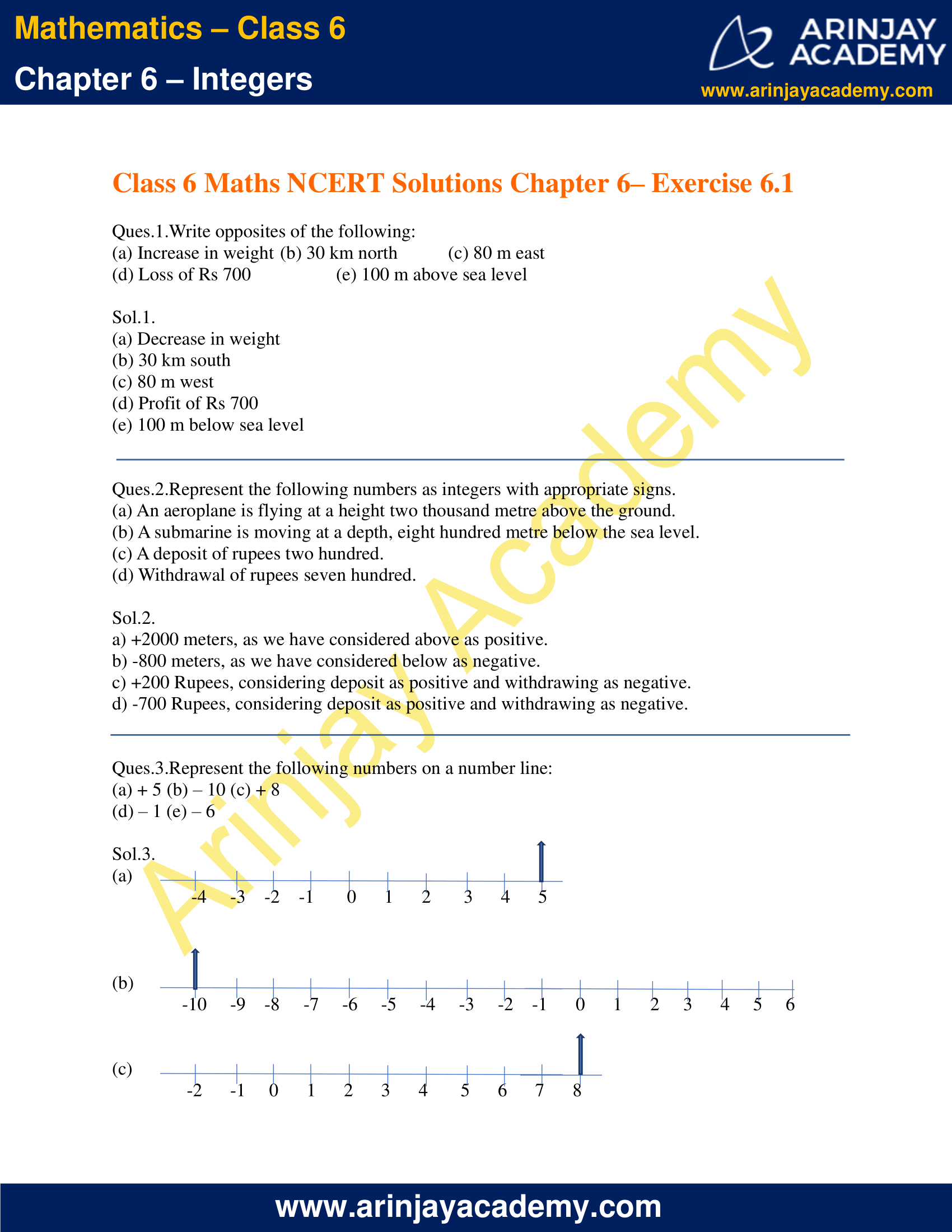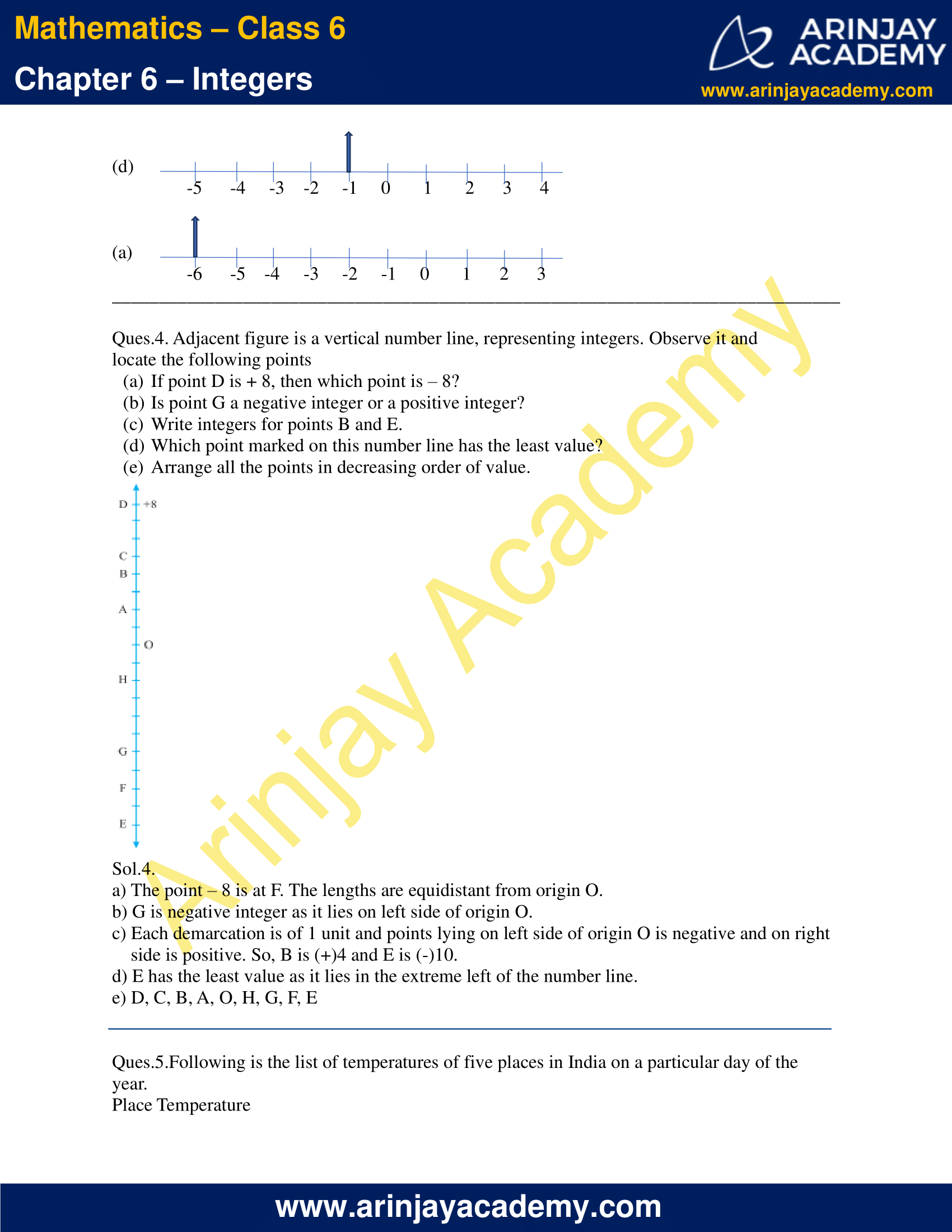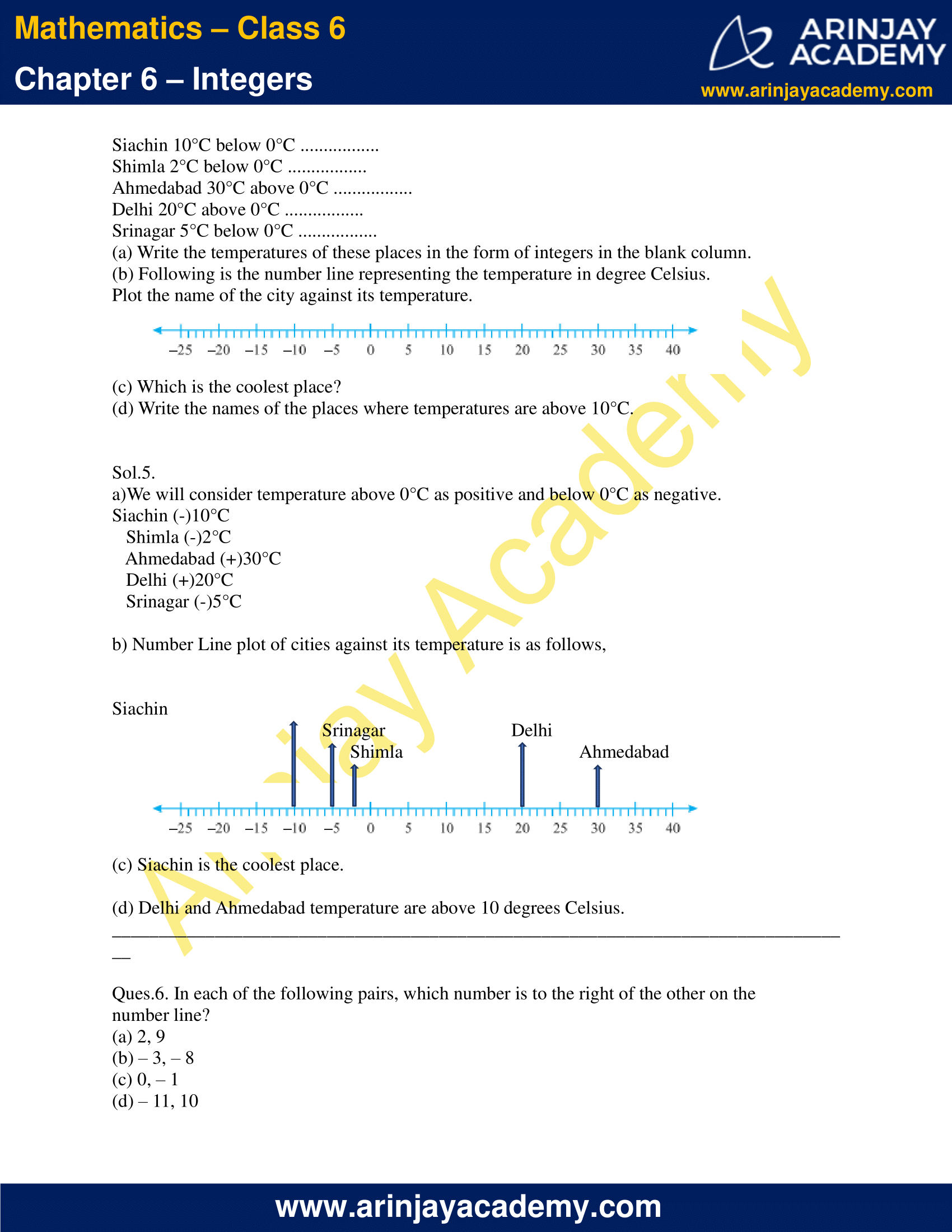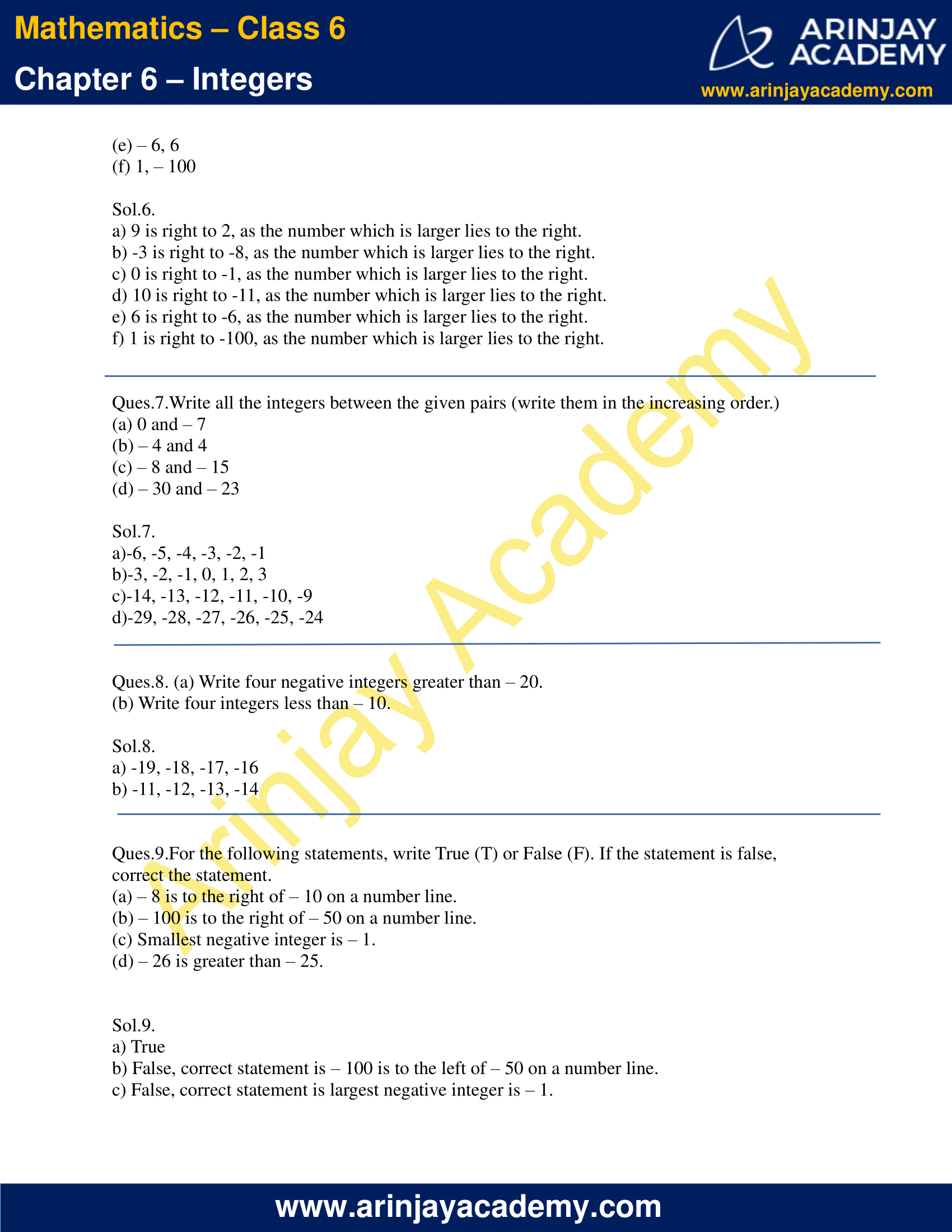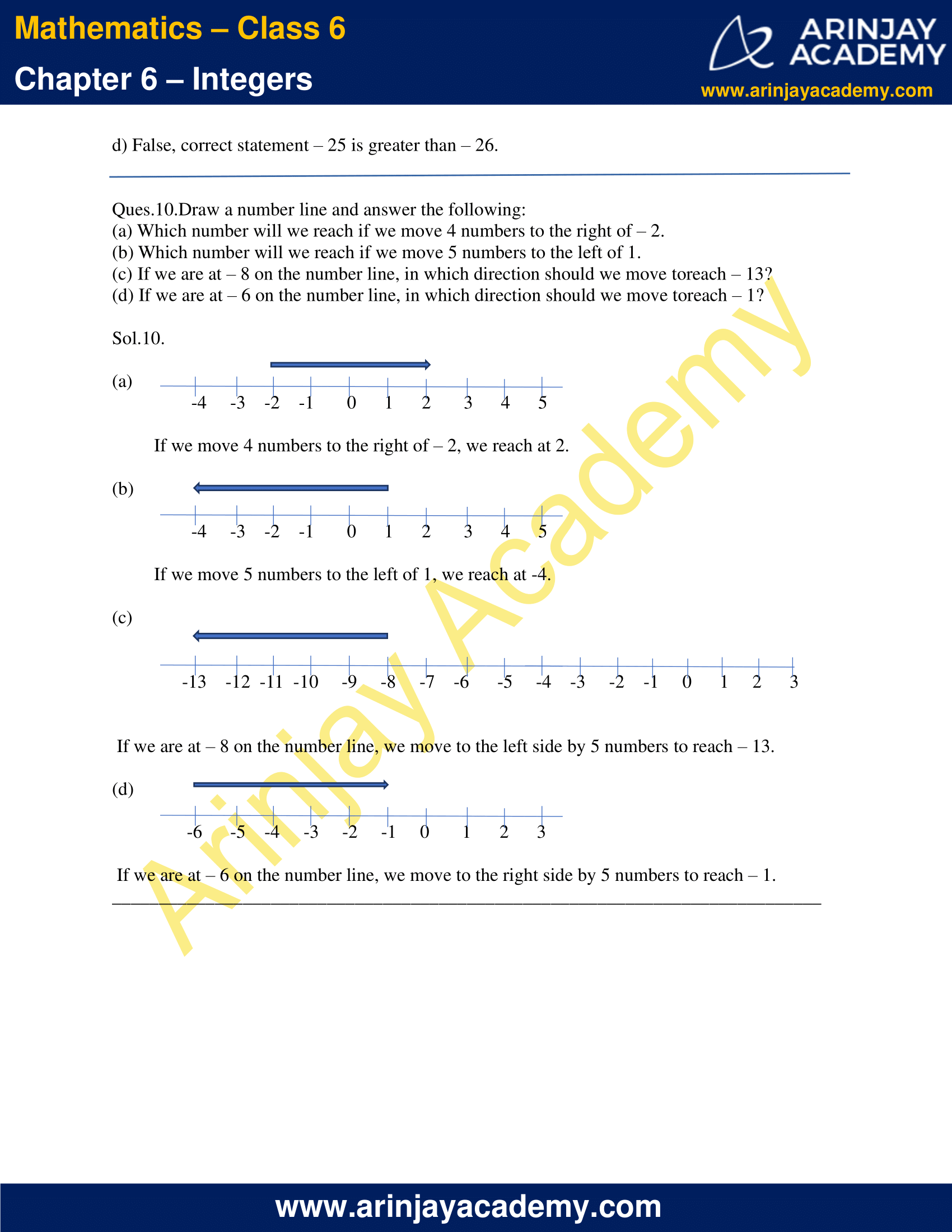### NCERT Solutions for Class 6 Maths Chapter 6 – Integers Exercise 6.2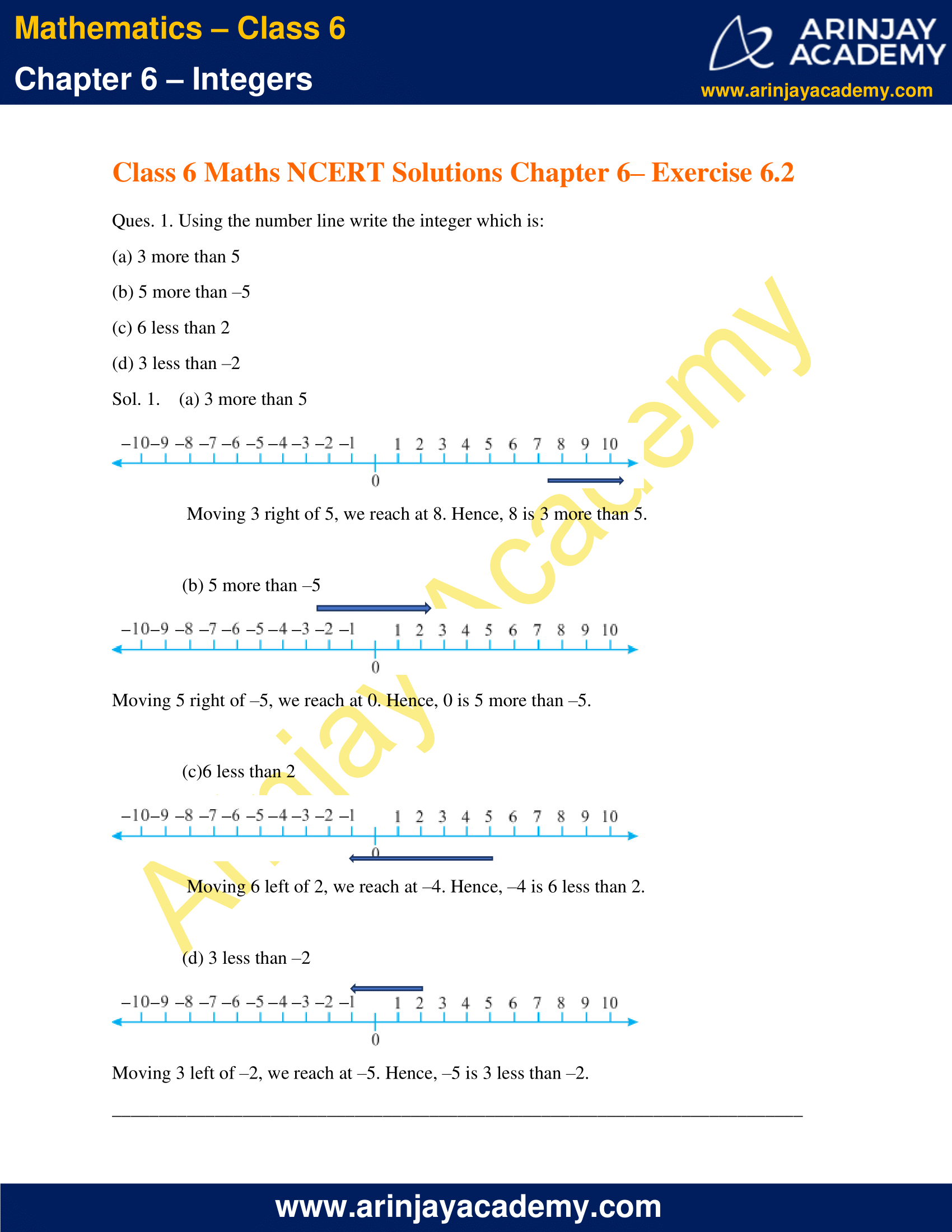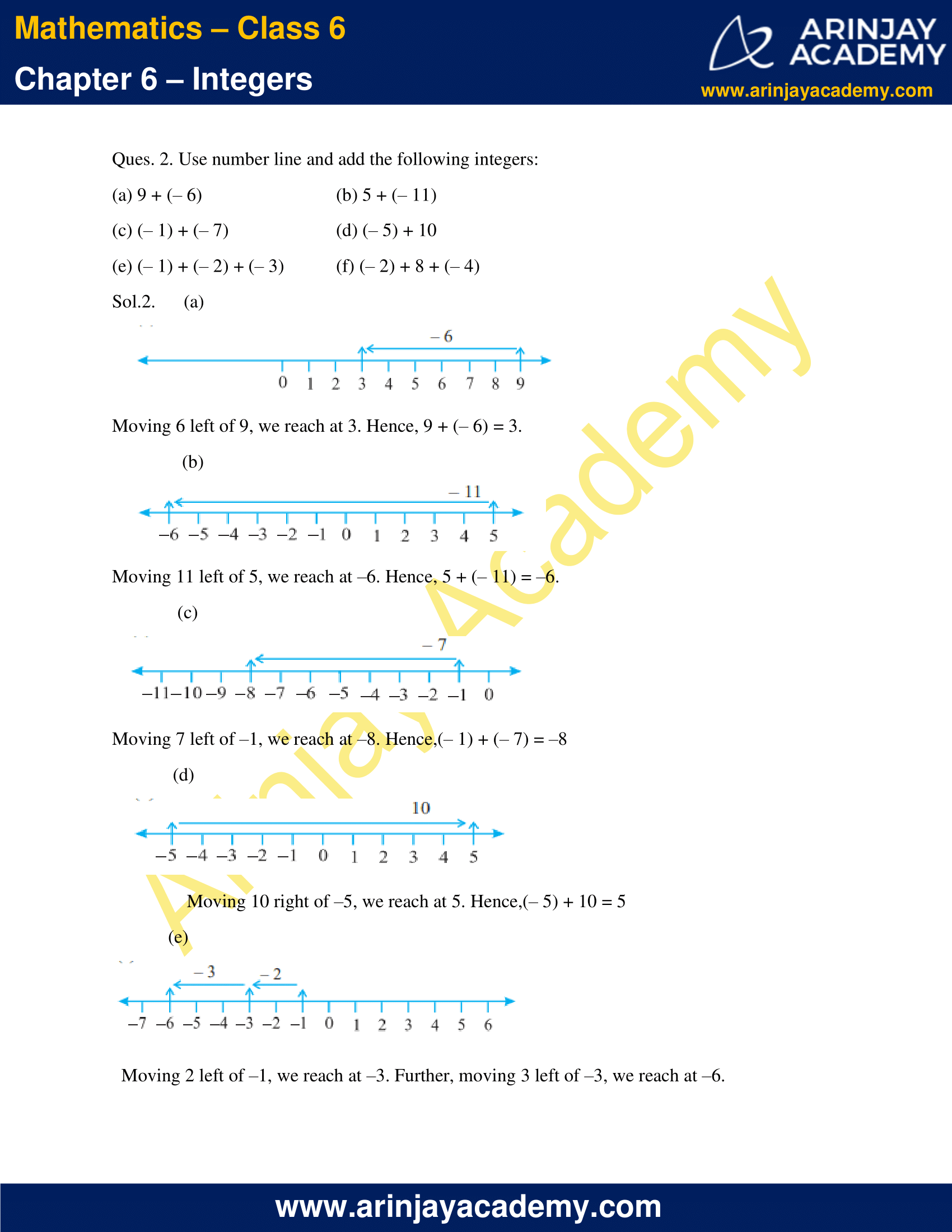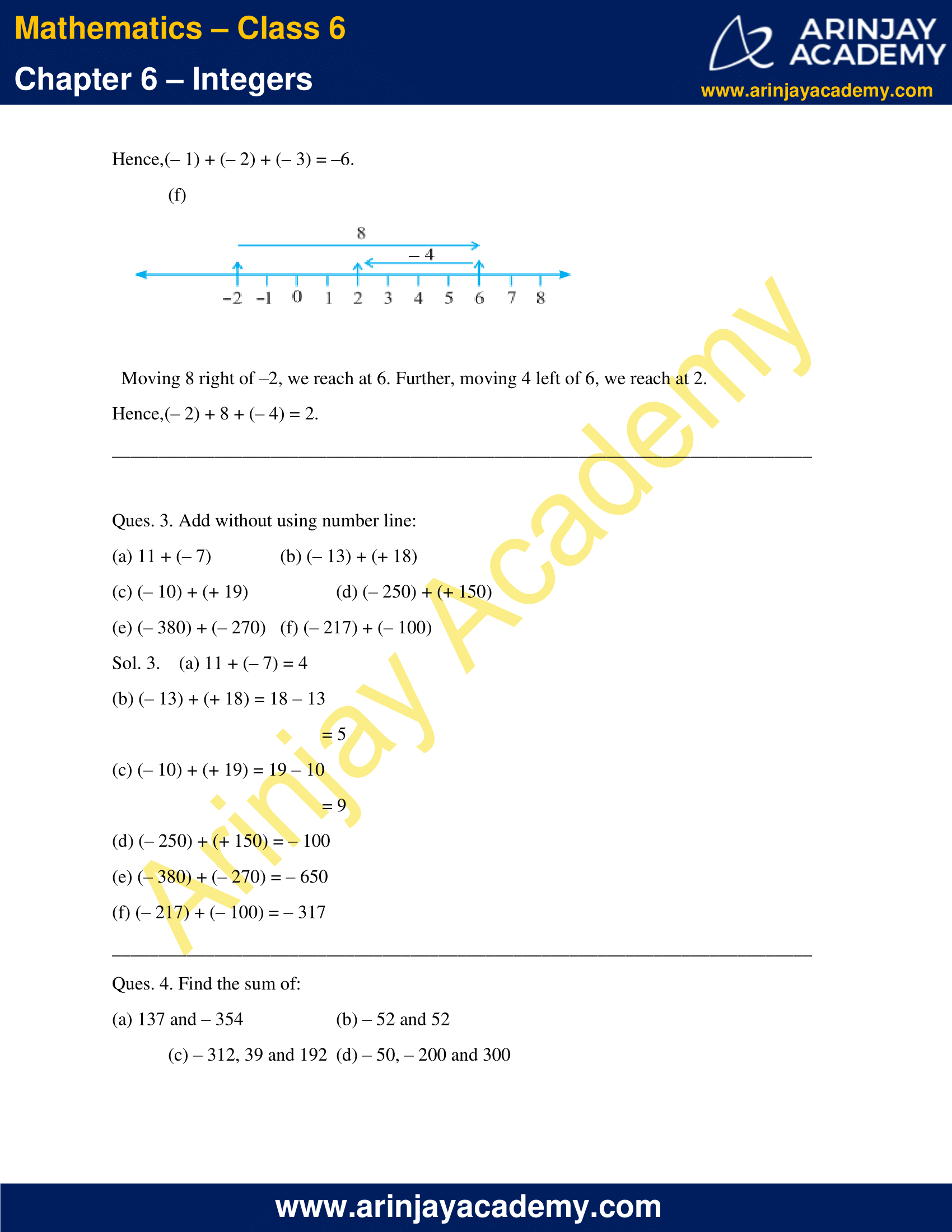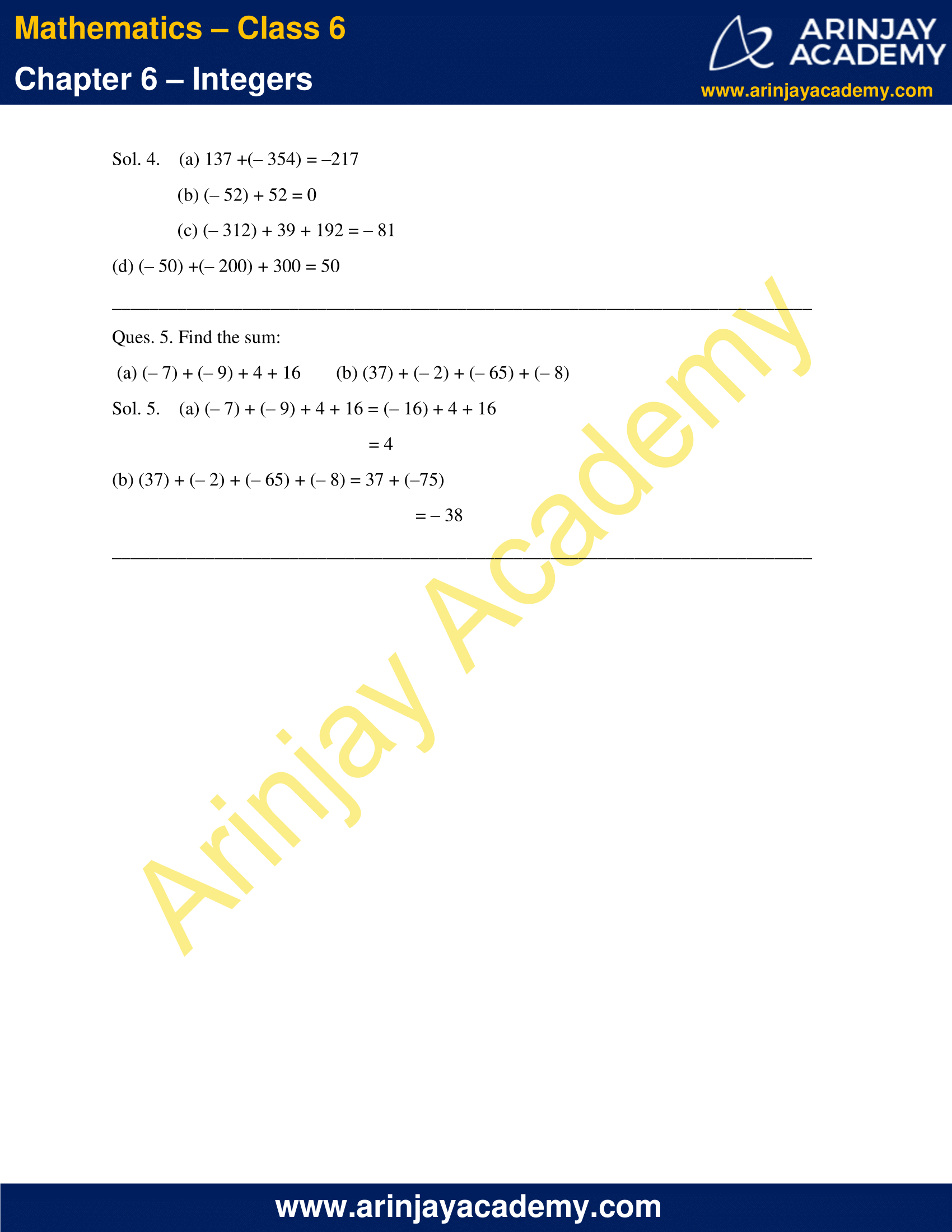### NCERT Solutions for Class 6 Maths Chapter 6 – Integers Exercise 6.3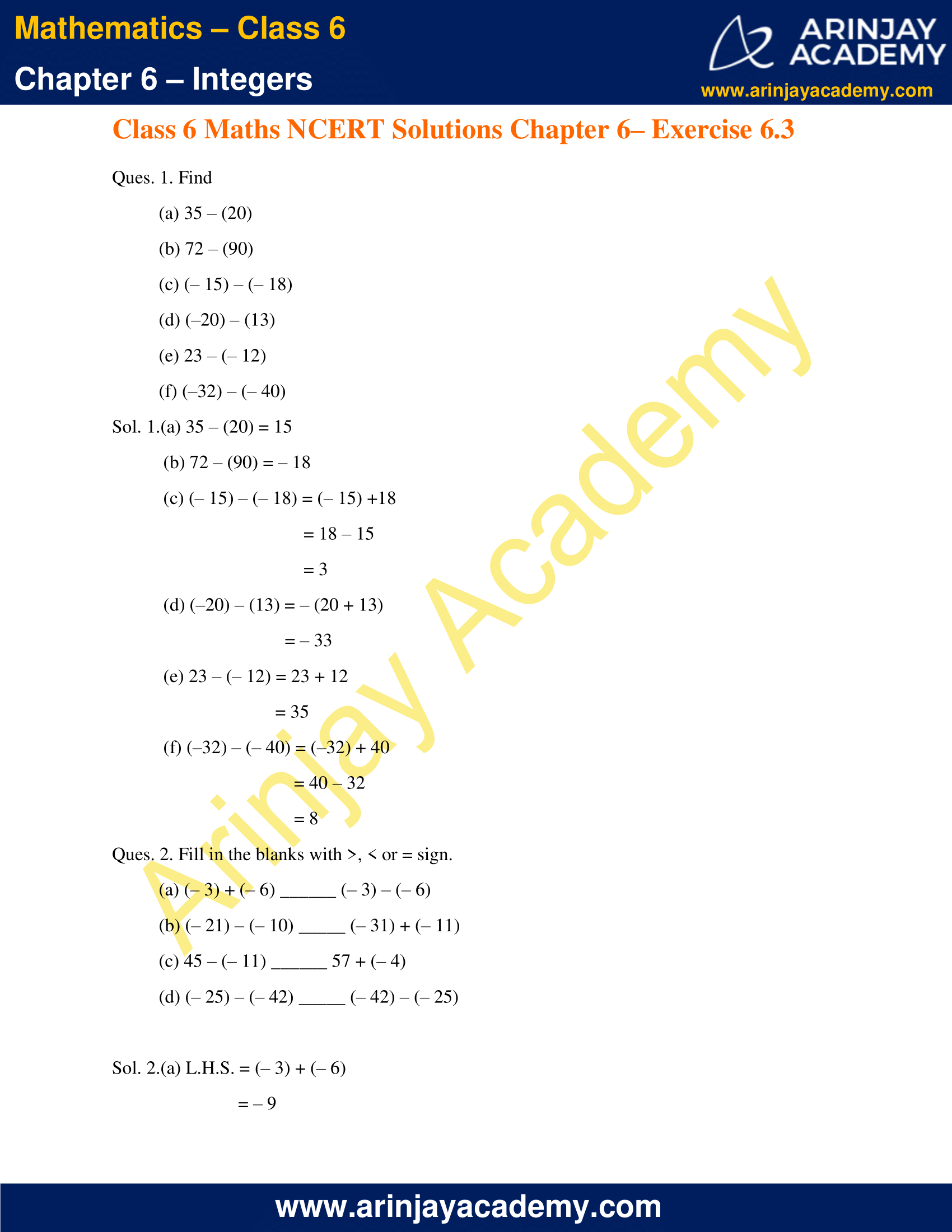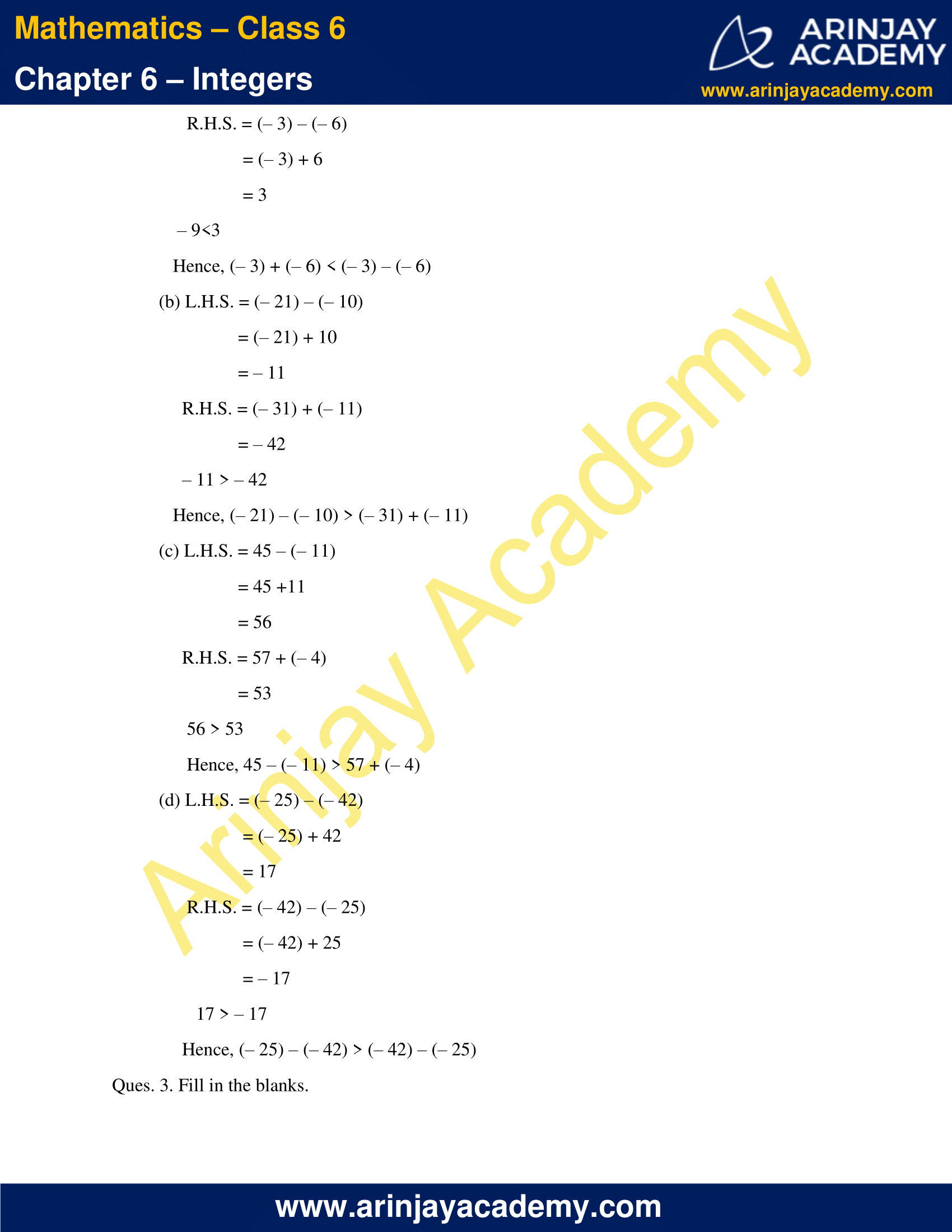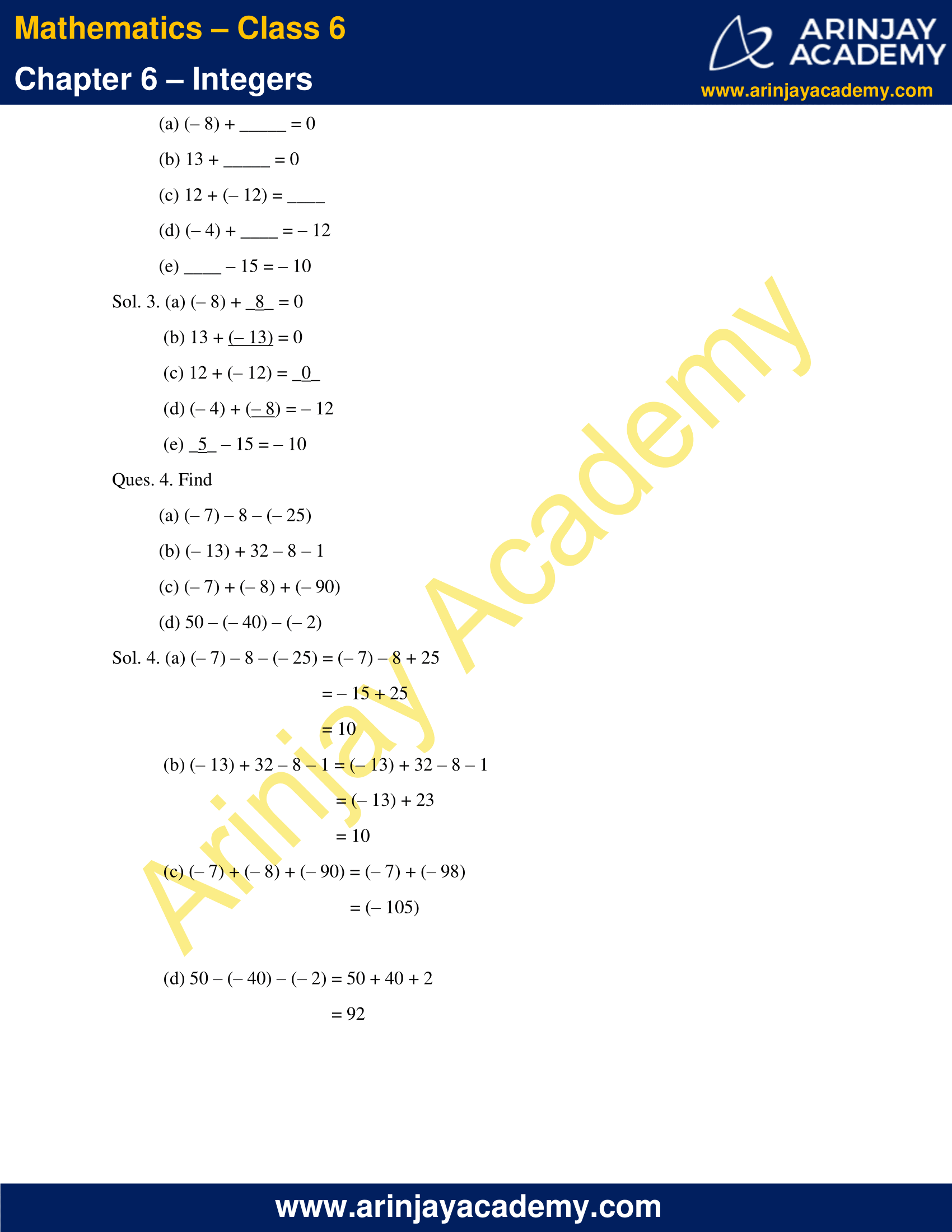### NCERT Solutions for Class 6 Maths Chapter 6 – Integers Exercise 6.1

Ques.1.Write opposites of the following:

(a) Increase in weight
(b) 30 km north
(c) 80 m east
(d) Loss of Rs 700
(e) 100 m above sea level

Sol.1.

(a) Decrease in weight
(b) 30 km south
(c) 80 m west
(d) Profit of Rs 700
(e) 100 m below sea level

Ques.2.Represent the following numbers as integers with appropriate signs.

(a) An aeroplane is flying at a height two thousand metre above the ground.
(b) A submarine is moving at a depth, eight hundred metre below the sea level.
(c) A deposit of rupees two hundred.
(d) Withdrawal of rupees seven hundred.

Sol.2.

a) +2000 meters, as we have considered above as positive.
b) -800 meters, as we have considered below as negative.
c) +200 Rupees, considering deposit as positive and withdrawing as negative.
d) -700 Rupees, considering deposit as positive and withdrawing as negative.

Ques.3.Represent the following numbers on a number line:

(a) + 5
(b) – 10
(c) + 8
(d) – 1
(e) – 6

Sol.3.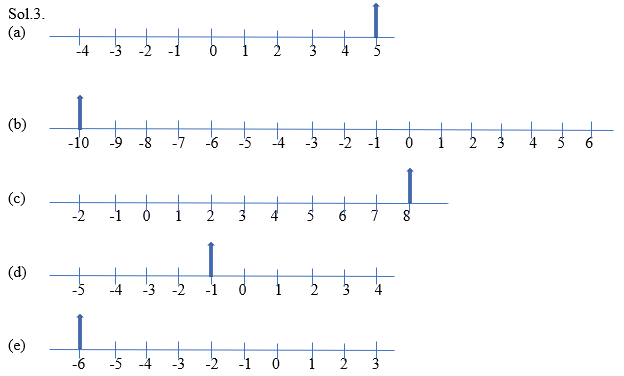Ques.4. Adjacent figure is a vertical number line, representing integers. Observe it and locate the following points

(a) If point D is + 8, then which point is – 8?
(b) Is point G a negative integer or a positive integer?
(c) Write integers for points B and E.
(d) Which point marked on this number line has the least value?
(e) Arrange all the points in decreasing order of value.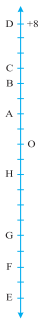Sol.4.

a) The point – 8 is at F. The lengths are equidistant from origin O.
b) G is negative integer as it lies on left side of origin O.
c) Each demarcation is of 1 unit and points lying on left side of origin O is negative and on right side is positive. So, B is (+)4 and E is (-)10.
d) E has the least value as it lies in the extreme left of the number line.
e) D, C, B, A, O, H, G, F, E

Ques.5.Following is the list of temperatures of five places in India on a particular day of the year.
Place Temperature
Siachin 10°C below 0°C ……………..
Shimla 2°C below 0°C ……………..
Delhi 20°C above 0°C ……………..
Srinagar 5°C below 0°C ……………..
(a) Write the temperatures of these places in the form of integers in the blank column.
(b) Following is the number line representing the temperature in degree Celsius.
Plot the name of the city against its temperature.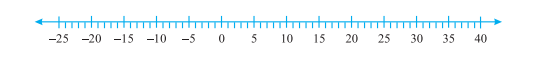(c) Which is the coolest place?
(d) Write the names of the places where temperatures are above 10°C.

Sol.5.

a) We will consider temperature above 0°C as positive and below 0°C as negative.
Siachin (-)10°C
Shimla (-)2°C
Delhi (+)20°C
Srinagar (-)5°C

b) Number Line plot of cities against its temperature is as follows,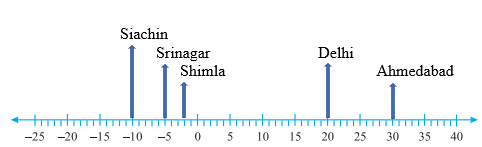(c) Siachin is the coolest place.

(d) Delhi and Ahmedabad temperature are above 10 degrees Celsius.

Ques.6. In each of the following pairs, which number is to the right of the other on the number line?

(a) 2, 9
(b) – 3, – 8
(c) 0, – 1
(d) – 11, 10
(e) – 6, 6
(f) 1, – 100

Sol.6.

a) 9 is right to 2, as the number which is larger lies to the right.
b) -3 is right to -8, as the number which is larger lies to the right.
c) 0 is right to -1, as the number which is larger lies to the right.
d) 10 is right to -11, as the number which is larger lies to the right.
e) 6 is right to -6, as the number which is larger lies to the right.
f) 1 is right to -100, as the number which is larger lies to the right.

Ques.7.Write all the integers between the given pairs (write them in the increasing order.)

(a) 0 and – 7
(b) – 4 and 4
(c) – 8 and – 15
(d) – 30 and – 23

Sol.7.

a)-6, -5, -4, -3, -2, -1
b)-3, -2, -1, 0, 1, 2, 3
c)-14, -13, -12, -11, -10, -9
d)-29, -28, -27, -26, -25, -24

Ques.8. (a) Write four negative integers greater than – 20.
(b) Write four integers less than – 10.

Sol.8.

a) -19, -18, -17, -16
b) -11, -12, -13, -14

Ques.9. For the following statements, write True (T) or False (F). If the statement is false, correct the statement.

(a) – 8 is to the right of – 10 on a number line.
(b) – 100 is to the right of – 50 on a number line.
(c) Smallest negative integer is – 1.
(d) – 26 is greater than – 25.

Sol.9.

a) True
b) False, correct statement is – 100 is to the left of – 50 on a number line.
c) False, correct statement is largest negative integer is – 1.
d) False, correct statement – 25 is greater than – 26.

Ques.10.Draw a number line and answer the following:

(a) Which number will we reach if we move 4 numbers to the right of – 2.
(b) Which number will we reach if we move 5 numbers to the left of 1.
(c) If we are at – 8 on the number line, in which direction should we move to reach – 13?
(d) If we are at – 6 on the number line, in which direction should we move to reach – 1?

Sol.10.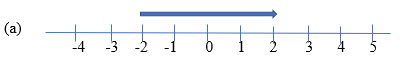If we move 4 numbers to the right of – 2, we reach at 2.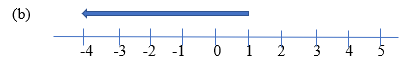If we move 5 numbers to the left of 1, we reach at -4.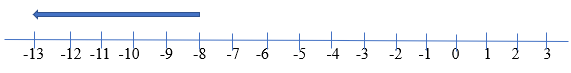If we are at – 8 on the number line, we move to the left side by 5 numbers to reach – 13.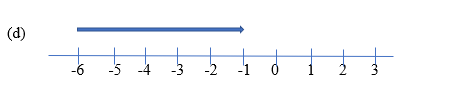If we are at – 6 on the number line, we move to the right side by 5 numbers to reach – 1.

### NCERT Solutions for Class 6 Maths Chapter 6 – Integers Exercise 6.2

Ques. 1. Using the number line write the integer which is:

(a) 3 more than 5
(b) 5 more than –5
(c) 6 less than 2
(d) 3 less than –2

Sol. 1.    (a) 3 more than 5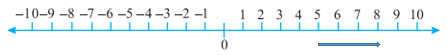Moving 3 right of 5, we reach at 8. Hence, 8 is 3 more than 5.

(b) 5 more than –5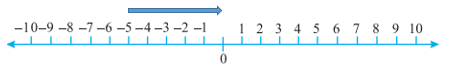Moving 5 right of –5, we reach at 0. Hence, 0 is 5 more than –5.

(c) 6 less than 2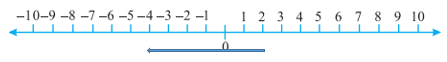Moving 6 left of 2, we reach at –4. Hence, –4 is 6 less than 2.

(d) 3 less than –2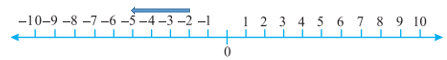Moving 6 left of 2, we reach at –4. Hence, –4 is 6 less than 2.

(d) 3 less than –2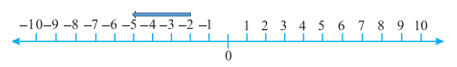Moving 3 left of –2, we reach at –5. Hence, –5 is 3 less than –2.

Ques. 2. Use number line and add the following integers:

(a) 9 + (– 6)
(b) 5 + (– 11)
(c) (– 1) + (– 7)
(d) (– 5) + 10
(e) (– 1) + (– 2) + (– 3)
(f) (– 2) + 8 + (– 4)

Sol.2. (a)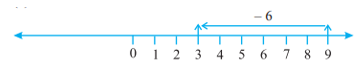Moving 6 left of 9, we reach at 3. Hence, 9 + (– 6) = 3.

(b)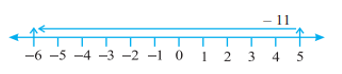Moving 11 left of 5, we reach at –6. Hence, 5 + (– 11) = –6.

(c)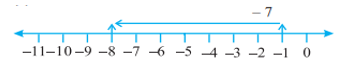Moving 7 left of –1, we reach at –8. Hence, (– 1) + (– 7) = –8

(d)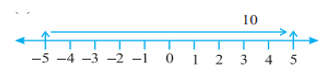Moving 10 right of –5, we reach at 5. Hence, (– 5) + 10 = 5

(e)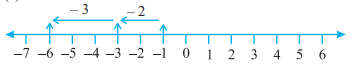Moving 2 left of –1, we reach at –3. Further, moving 3 left of –3, we reach at –6.

Hence, (– 1) + (– 2) + (– 3) =  –6.

(f)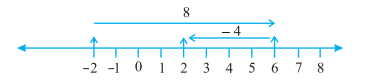Moving 8 right of –2, we reach at 6. Further, moving 4 left of 6, we reach at 2.

Hence, (– 2) + 8 + (– 4) = 2.

Ques. 3. Add without using number line:

(a) 11 + (– 7)
(b) (– 13) + (+ 18)
(c) (– 10) + (+ 19)
(d) (– 250) + (+ 150)
(e) (– 380) + (– 270)
(f) (– 217) + (– 100)

Sol. 3.    (a) 11 + (– 7) = 4
(b) (– 13) + (+ 18) = 18 – 13 = 5
(c) (– 10) + (+ 19) = 19 – 10 = 9
(d) (– 250) + (+ 150) = – 100
(e) (– 380) + (– 270) = – 650
(f) (– 217) + (– 100) = – 317

Ques. 4. Find the sum of:

(a) 137 and – 354
(b) – 52 and 52
(c) – 312, 39 and 192
(d) – 50, – 200 and 300

Sol. 4.    (a) 137 + (– 354) = –217
(b) (– 52) + 52 = 0
(c) (– 312) + 39 + 192 = – 81
(d) (– 50) + (– 200) + 300 = 50

Ques. 5. Find the sum:

(a) (– 7) + (– 9) + 4 + 16
(b) (37) + (– 2) + (– 65) + (– 8)

Sol. 5. (a) (– 7) + (– 9) + 4 + 16 = (– 16) + 4 + 16 = 4
(b) (37) + (– 2) + (– 65) + (– 8) = 37 + (–75) = – 38

### NCERT Solutions for Class 6 Maths Chapter 6 – Integers Exercise 6.3

Ques. 1. Find

(a) 35 – (20)
(b) 72 – (90)
(c) (– 15) – (– 18)
(d) (–20) – (13)
(e) 23 – (– 12)
(f) (–32) – (– 40)

Sol. 1.(a) 35 – (20) = 15

(b) 72 – (90) = – 18

(c) (– 15) – (– 18) = (– 15) +18
= 18 – 15
= 3

(d) (–20) – (13) = – (20 + 13)
= – 33

(e) 23 – (– 12) = 23 + 12
= 35

(f) (–32) – (– 40) = (–32) + 40
= 40 – 32
= 8

Ques. 2. Fill in the blanks with >, < or = sign.

(a) (– 3) + (– 6) ______ (– 3) – (– 6)
(b) (– 21) – (– 10) _____ (– 31) + (– 11)
(c) 45 – (– 11) ______ 57 + (– 4)
(d) (– 25) – (– 42) _____ (– 42) – (– 25)

Sol. 2.(a) L.H.S. = (– 3) + (– 6)
= – 9
R.H.S. = (– 3) – (– 6)
= (– 3) + 6
= 3
– 9<3
Hence, (– 3) + (– 6) < (– 3) – (– 6)

(b) L.H.S. = (– 21) – (– 10)
= (– 21) + 10
= – 11
R.H.S. = (– 31) + (– 11)
= – 42
– 11 > – 42
Hence, (– 21) – (– 10) > (– 31) + (– 11)

(c) L.H.S. = 45 – (– 11)
= 45 +11
= 56
R.H.S. = 57 + (– 4)
= 53
56 > 53
Hence, 45 – (– 11) > 57 + (– 4)

(d) L.H.S. = (– 25) – (– 42)
= (– 25) + 42
= 17
R.H.S. = (– 42) – (– 25)
= (– 42) + 25
= – 17
17 > – 17
Hence, (– 25) – (– 42) > (– 42) – (– 25)

Ques. 3. Fill in the blanks.

(a) (– 8) + _____ = 0
(b) 13 + _____ = 0
(c) 12 + (– 12) = ____
(d) (– 4) + ____ = – 12
(e) ____ – 15 = – 10

Sol. 3. (a) (– 8) + _8_ = 0
(b) 13 + (– 13) = 0
(c) 12 + (– 12) = _0_
(d) (– 4) + (– 8) = – 12
(e) _5_ – 15 = – 10

Ques. 4. Find

(a) (– 7) – 8 – (– 25)
(b) (– 13) + 32 – 8 – 1
(c) (– 7) + (– 8) + (– 90)
(d) 50 – (– 40) – (– 2)

Sol. 4. (a) (– 7) – 8 – (– 25) = (– 7) – 8 + 25
= – 15 + 25
= 10

(b) (– 13) + 32 – 8 – 1 = (– 13) + 32 – 8 – 1
= (– 13) + 23
= 10

(c) (– 7) + (– 8) + (– 90) = (– 7) + (– 98)
= (– 105)

(d) 50 – (– 40) – (– 2) = 50 + 40 + 2
= 92

With this we come to the end of NCERT Solutions for Class 6 Maths Chapter 6 Integers. We hope these helped you study your subject.